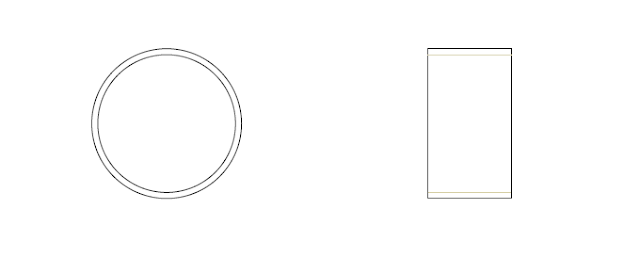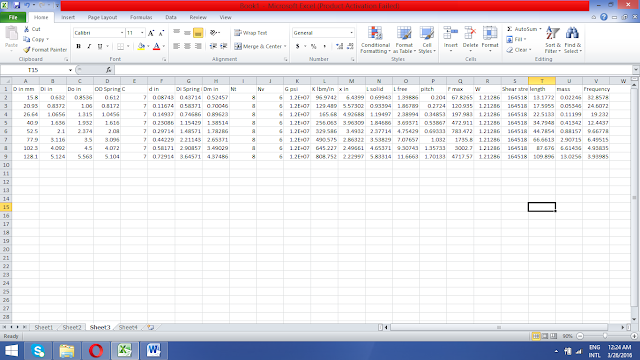# Design a Projectile LauncherDesign a spring-loaded gun to shoot a projectile to your worst enemy. The conceptual design of this gun is shown on the next page.
It consists of a commercially available pipe (1),
bottom cap (2)
with an opening for the trigger pin, trigger pin (3),
piston (4),
with a machined groove (6) to engage the trigger pin,
nut (7),
projectile (8),
helical spring (9)
and  aerodynamic projectile nose (10) of negligible weight.

The projectile has a mass of 0.5 lbm. The target is located on the ground and 250 ft away. The gun will shoot from the ground level at an angle that maximizes the horizontal reach of the projectile. The spring must be preloaded (i.e. compressed) in order to install inside the gun and provide an initial compressive force. The force required to pull the trigger pin should be less than 50 lbf.

The spring is compressed to solid length by tightening the nut with a power wrench. The trigger pin is inserted through the bottom cap and into the groove to secure the gun in the loaded position. The nut is then backed up all the way and the trigger pin pulled by hand to shoot.

Specify all design parameters listed below. Make all necessary choices and assumptions, which should be listed along with your calculations. Use sound engineering judgement. Provide a professionally written calculation package and schematics to get full credit. Use Excel, MathCAD or MathLab to facilitate design iterations and provide detailed screenshots of inputs and outputs. Comply with all notes listed on the cover page.

1) Projectile velocity and angle when exiting the gun.
2) Stroke or distance traveled by the piston to shoot.
3) Distance that the spring should be preloaded or compressed when installed.
4) Outside diameter (OD) and length of the projectile knowing that it’s made of aluminum 7075-T6 tubing having 1/8” wall thickness.
5) Diametral clearances between pipe and spring, and between pipe and projectile.
6) Internal diameter (ID) of the pipe.
7) Mean diameter, wire diameter, material choice, spring constant, spring index, number of active coils, total number of coils, solid length, free length, pitch, maximum spring force, minimum spring force, maximum stress, static safety factor, critical length, weight, natural frequency and fatigue safety factor of the spring based on Gerber.
8) If necessary, consider the use of nested springs, in which case, all the parameters listed in item 7) should be specified for each spring.
9) Nominal diameter, thread type and material choice for the threaded rod.
10) Rod groove dimensions.
11) Safety factor against tensile yielding of the rod thread and rod body.
12) Use your knowledge of dynamics to estimate the force generated on the nut upon impact and to relate projectile velocity to spring constant.
13) Safety factor against shearing the rod and nut threads.
14) Torque required to turn the nut in order to load the gun.
15) Force to pull the trigger pin.
16) Safety factor against shearing the trigger pin.
17) Gerber fatigue safety factor for the rod.

Solution

# Projectile

To specify the dimensions of projectile, dimensions of pipe should be known. Schedule STD was randomly selected from the available standards schedules and pipe with various diameter range from 0.5 in to 5 in (nominal diameter) where examine and result are shown below

From the equation that density is equal to mass per unit volume, length and diameter of projectile can be selected.

L×t×(Douter-t)= ρ×π/m

Here diameter of the projectile should be kept 0.02 in less than the inner diameter of pipe for diametrical clearance and by this length can find out.Outer diameter and length of the projectile is taken as 3.096 in and 1.72 in reason will be explain in lateral discussion. Internal diameter of the pipe is 3.5 in.

To have the maximum range at a given velocity angle of projection should be 45 degree. So to have 250ft as maximum range angle should be 45 degrees and by using following formula, velocity of the projectile can be find out.

R=  (v^2×sin⁡〖2∝〗)/g
v = 89.68 ft/sec
Following equation show the relation between projectile velocity and spring constant
v= √((k ×x^2)/m)
Form this equation we can develop a relation
k ×x^2  = v^2  ×m = 4021.75

Spring design
While designing spring following formulas, consideration and assumptions were taken

Diametrical clearance = 0.02 in
Spring constant = 7
D mean = C ×d
D inner = D mean – d
K = G×d^4/(8D^3 N)
Total number of coils Nt = active coils Nv + 2
Free length = 2×solid length
Solid length = wire diameter  ×  Nt
Pitch = (free length-2d)/Nv
Force F max  = K ( free length – solid length)
Shear stresses in spring τ=8WDF/(πd^3 )
Factor of safety = Allowable stresses/ design stresses

Using above formulas calculations we carried out using different outer diameter with respect to pipe diameter, different wire diameter, different number of totals coils and different number of active coils.From those entire calculations one with red mark is selected because this one set best with our pipe, required length of springs, number of coils and it provide sufficient force to projectile required velocity.
Output
Outer diameter of spring = 3.5 in
Inner diameter of spring = 2.211 in
Sprig index = C = 7
Wire diameter = d = 0.443 in
Mean diameter = Dm = 2.65 in
Nt = 6
Nv = 4
Shear modulus = G = 11500000 psi
K = 735.863 lbm/in
Compression distance = x = 2.34 in
Solid length = 2.65 in
Free length = 5.30 in
Pitch = 1.10 in
Force = 1952.77 lbf
Shear stresses = 185083 psi
Mass = 2.18 lbm
Frequency = 9.18 Hz
FOS = 175000000/185083 = 5.40
Distance travel by the piston is equal to the compression distance of the spring equal to 2.34 in. preloading is done to make the nut and bolt tight and for this compression of 0.1 in will be enough.

Threaded rod should be the one that can fit inside the spring and provide the sufficient power to compress the spring. From standard tables following Acme square threads were selected and analyzed for stresses and torque. Material of the rod is steel.

For Rod
Force on the rod is F = 1952 (spring force)
Steel yield point = 31200 psi
Area of rod = (π×d^2)/4 = 3.14 in^2
Stresses = force/area = 621 psi
FOS = yield stresses/stresses = 50.24

Force on the rod is F = 1952 (spring force)
Steel yield point = 31200 psi
Area of threads from table = 1.824 in^2
Stresses = force/area = 1070.17 psi
FOS = yield stresses/stresses = 29.15
Torque requirement
As d = 2 in
Dm = 2 – pitch/2 = 1.875 in
Friction coefficient = 0.15 steel dry
Torque required to compresses the spring
T_r=  (F×dm)/2  ×(l+πfdm)/(πdm-fl)
T_r=434 lbfin
Maximum nominal shear stress in threads
τ =3F/(π d_r Nt ×pitch)
d_r=1.75 in
τ=2131.39 psi
Dividing this shear stress with torsional yield stresses 7800 psi we have FOS = 3.65

Rod groove dimension
Steel shear strength = 15600 psi
After the factor of safety = 2
Shear strength = 7800 psi
Shear Stress = force/area

So

Area = force / shear stress = 0.25 in^2
Area of square = ab = 2a = 0.25 in ^2
a = 0.125 in
Depth of groove should also be 0.125 in to compensate stresses.Trigger Pin

Let pin is made of steel and its opening cavity is also made of steel. Friction between steel and steel is 0.42. Forces required to remove the pin is F =μ N where N is force applied by the spring so F = 819.84 lbf. As trigger pin made of the same dimension as groove on rod so the safety of factor for it will be same that is 2.

Nut
As nut is also made of steel and has same thread dimensions as screw has so the torque and FOS for him is as follow.
τ =3F/(π d_r Nt ×pitch)
d_r=2 in
τ=1864 psi
Dividing this shear stress with torsional yield stresses 7800 psi we have FOS = 4.18
Upon impact of nut with wall of launcher force which act of nut is same force which spring has in it when it was in compresses form. Upon releasing same force will act on the nut when it hit the wall.